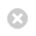Close

Quantum Spin Dynamics

Home# Teaching

This page lists some resources for lectures course John Morton currently teaches, or has taught in the past.

### ELEC1001: Electronic Circuits (UCL, 2012-)

UCL students, please use Moodle to access all up-to-date lecture materials for this course

### Magnetic Properties of Materials (Oxford University, 2010-2012)

Lecture notes Part I, and the equations

Lecture notes Part II, and the equations

Lecture notes Part III, and the equations

Problem Sheet and the Solutions

### Electrical and Optical Properties of Materials (Oxford University, 2009-2011)

Lecture notes Part I, and the equations

Lecture notes Part II, and the equations

Lecture notes Part III, and the equations

Lecture notes Part IV, and the equations

Lecture notes Part V, and the equations

Problem Sheet

Please let me know of any errors you spot in these materials, so they can be corrected.